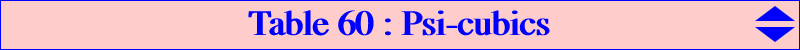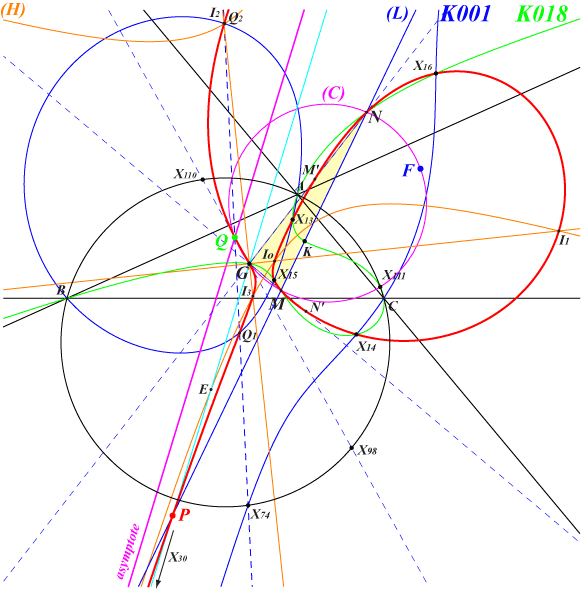A Psi-cubic is a cubic invariant under the transformation Psi described here. See also Table 48. Any such cubic must pass through X(2) and the circular points at infinity which are the singular points of Psi. This is a necessary but not sufficient condition. Only two circum-cubics are Psi-cubics namely K018 and K022. Both must contain the vertices of the second Brocard triangle, the Psi-images of A, B, C. The following table gives a selection of Psi-cubics with singular focus F, infinite point S.cubic X(i) on the cubic for i = F S remarks K018 2, 6, 13, 14, 15, 16, 111, 368, 524, 5000, 5001, 11537, 11549, 32618, 32619, 39162, 39163, 39164, 39165, 47072, 47073 X(111) X(524) isogonal focal nK0 K022 2, 3, 110, 525, 7473, 35910, 35911, 35912 X(14649) X(525) nK K048 2, 15, 16, 511, 1113, 1114, 7709, 13414, 13415, 47638 X(2) X(511) focal K193 2, 15, 16, 512, 3111, 9147 X(2) X(512) focal K463 2, 3, 15, 16, 30, 110, 5463, 5464, 39162, 39163, 39164, 39165 X(110) X(30) focal K508 2, 13, 14, 15, 16, 30, 98, 476, 1316, 18332, 41330 X(2) X(30) focal K733 2, 13, 14, 523, 14932, 14995 X(9140) X(523) K816 2, 3, 4, 74, 110, 125, 131, 541, 11472, 14685, 39162, 39163, 39164, 39165 X(14685) X(541) focal K874 2, 4, 13, 14, 125, 542, 14847, 46465, 46466 X(2) X(542) focal, see Table 59 K881 2, 6, 13, 14, 15, 16, 30, 111, 1995, 2549, 5913, 14899, 35607, 35608, 35609 X(5653) X(30) K882 2, 13, 14, 15, 16, 30, 184, 186, 3165, 3166, 39242 X(14696) X(30) K883 2, 3, 13, 14, 15, 16, 30, 110, 399 X(9138) X(30) K884 2, 4, 13, 14, 15, 16, 30, 113, 125, 5667, 9730, 16319, 24007, 24008, 46465, 46466 X(14697) X(30) K885 2, 5, 13, 14, 15, 16, 30, 265, 568, 3448, 10277 X(14698) X(30) K886 2, 3, 6, 110, 111, 115, 542, 6792, 14899, 35607, 35608, 35609 X(9178) X(542) K887 2, 6, 23, 111, 182, 187, 353, 381, 6032, 9140, 10546, 14899, 35607, 35608, 35609 X(14699) X(11645) K888 2, 6, 23, 111, 182, 184, 186, 187, 353, 11649, 51818 X(15755) X(11649) K889 2, 100, 187, 353, 739, 1083, 1340, 1341, 5638, 5639, 11650, 11651, 11652 X(15756) ? K890 2, 184, 186, 187, 353, 1340, 1341, 3148, 5638, 5639, 11653, 13509, 14917, 51818 X(15757) X(14917) K891 2, 187, 353, 538, 1340, 1341, 3117, 5638, 5639, 8289, 11654, 11655 X(15758) X(538) K893 2, 3, 6, 110, 111, 542, 2482, 14916, 39162, 39163, 39164, 39165 X(3) X(542) focal K1141 2, 6, 111, 523, 5653, 5912, 5968, 14899, 35606, 35607, 35608, 35609 X(11579) X(523) K1142 2, 4, 6, 25, 111, 112, 125, 1560, 5622, 14899, 35607, 35608, 35609, 35901, 35902, 35903, 35904, 36201, 36202, 36203, 36204 X(35905) X(36201) K1286 2, 3, 23, 110, 182, 187, 353, 9829, 11645, 15080, 39162, 39163, 39164, 39165, 51797, 51798, 51799, 51800 X(51800) X(11645) K1295 2, 3, 110, 352, 511, 574, 599, 7998, 8724, 10717, 10989, 11178 39162, 39163, 39164, 39165 X(8724) X(511) focal K1296 2, 3, 5, 13, 14, 110, 399, 3448, 6592, 39162, 39163, 39164, 39165 ? ? focalFermat Psi-cubics The Fermat Psi-cubics (orange cells) are those of the pencil with basis points X(2), X(13), X(14), X(15), X(16), X(30), the circular points at infinity and with tangent at X(2) passing through X(98), X(110) unless the cubic splits into the line at infinity and the two lines passing through X(2) and the Fermat points X(13), X(14). The Fermat Psi-cubic K(P) that passes through P on the Euler line obviously contains Q = Psi(P) on the line X(2), X(98), X(110), etc. Q is the intersection of the cubic with its real asymptote. Let (L) be the line passing through X(6), P and let (C) be the circle which is its Psi-image. (L) and (C) meet at two (not always real) points M, N on K018. K(P) is the isogonal pK with pivot X(30) with respect to the triangle T whose vertices are G, M, N. It follows that the singular focus F of K(P) is the antipode of Q on the circumcircle of T. F lies on the line X(2), X(690), etc. Hence there is only one proper focal cubic in this pencil and this is K508. Moreover, K(P) must pass through the in/excenters of T which are the common points of the axes of the Steiner ellipses and the polar conic of X(30) in K(P), the rectangular hyperbola (H) passing through X(30), X(395), X(396), X(523) and the midpoint E of GP. These in/excenters are the centers of anallagmaty of K(P) and the tangents at these points are parallel to the Euler line of ABC. The points M' = X(30)M /\ X(2)N and N' = X(30)N /\ X(2)M obviously lie on K(P). K(P) meets the Neuberg cubic K001 at seven fixed points namely X(13), X(14), X(15), X(16), X(30), the circular points at infinity. The two remaining common points Q1, Q2 lie on the line passing through X(74) and Q = Psi(P) hence they must be X(30)-Ceva conjugates. It follows that they also must lie on the conic passing through the X(30)-Ceva conjugate of Psi(P), X(2132), the vertices of the cevian triangle of X(30). These four latter points lie on K001.Special cases • P = X(2) : K(P) splits into the three lines mentioned above. • P = X(30) : the real asymptote is the Euler line, the singular focus is X(9185).Psi-pivotal cubics The Psi-pivotal cubics (blue cells) are cubics obviously invariant under Psi. Indeed, for P ≠ G, the cubic K(P) is the locus of M such that P, M, Psi(M) are collinear. Hence, K(P) can be seen as an isogonal pivotal cubic in the non proper triangle with vertices X(2) and the circular points at infinity. K(P) is a focal cubic with focus F = Psi(P). It contains the singular points of Psi (G, two circular points at infinity) and its fixed points (the four foci of the Steiner inellipse). It follows that these cubics are in a same net. K(P) is also the locus of the common points of a line passing through P and the circle passing through G, F which is its Psi-image. Also, K(P) is the locus of the contacts of tangents drawn through F to the circles passing through G, P. When P lies on the Brocard axis, F lies on the Parry circle and all the cubics are in a same pencil and pass through X(15), X(16). When P lies on the Fermat axis, F lies on the circle passing through X(2), X(13), X(14), X(111), X(476) and all the cubics are in a same pencil and pass through X(13), X(14). With P = X(6) which is the intersection of the two previous axes, we obtain K018 with singular focus X(111).